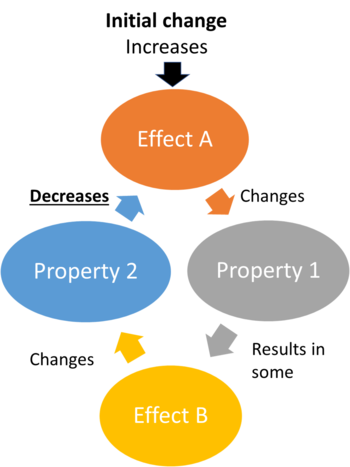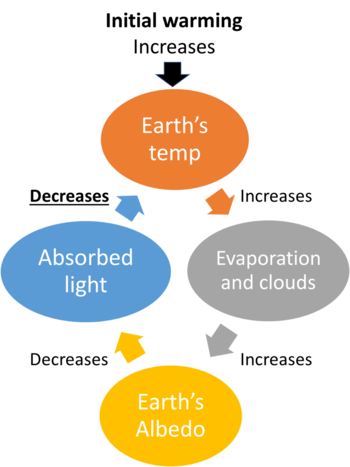# Negative feedbackFigure 1. A flow chart to show the general process of some negative feedback, with an initial "push" to the system causing other effects that keep the system in its original state.

Negative feedback is a process that minimizes or reduces some initial effect, generally some disturbance causes some secondary effect that in turn minimizes the magnitude of the initial disturbance. This causes some initial change to grow smaller, keeping the system from moving out of its equilibrium state. The flow chart shown in Figure 1 can be used to clarify how exactly these changes occur, how they are connected, and how they result in an overall decrease of some initial effect. Note that every time the cycle repeats the effect gets weaker. The word 'negative' doesn't mean this is a bad feedback, it means that it's pointing in the opposite direction as the initial change (like a negative sign, which is where the term comes from).

From Figure 1, the explanation of what a negative feedback is can be simplified. Essentially, it is some initial push being applied to a system, creating some effect A. This effect can then change some properties throughout the system that then produces some later effect B, which may continue to change properties of the system. Eventually, the effect B then reduces the severity of the initial effect A. This pushes a system back into equilibrium after some disturbance. There are numerous examples of negative feedback in mechanics, in the human body, and in nature. Additionally, the concept of negative climate feedbacks are important in understanding climate change.

## Examples of Negative FeedbackFigure 2. A flow chart showing the one of the most significant negative feedback loops in the climate system - the cloud negative feedback.

To simplify what this idea of a negative feedback actually means, it sometimes helps to approach the concept in a variety of different contexts. Some examples of negative feedback loops that are simple to understand are:

• The way that the body cools itself. As internal body temperature rises sweat is produced. The sweat then evaporates, cooling the skin and returning the body temperature to a more manageable level.
• In mechanical systems, the motion of a spring can be seen as a negative feedback. When a spring is compressed and released, it will return to its initial shape after the force on it is gone. The tendency of the spring is to move back to its equilibrium position after being compressed.
• As well, when a student receives a test back where incorrect answers have been marked, it is a type of negative feedback. By seeing which questions were done incorrectly, the student determines how to do the problems correctly and the questions will be done correctly on the next test.

One example of a negative feedback in the climate is the formation of clouds - and corresponding reduction in solar radiation absorption. This cloud feedback is outlined in Figure 2, and is one of the most important negative feedbacks in the climate system. As the surface temperature of the Earth increases there are increased levels of evaporation from the oceans. This increased evaporation results in more clouds forming in the lower atmosphere. These clouds in turn reflect some incident solar radiation back into space, slightly decreasing the surface temperature, reducing the number of clouds.